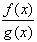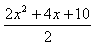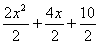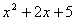Dividing
Example 2

Given f(x) = 2x2 + 4x + 10 and g(x) = 2, find.

Step 1. Replace f(x) and g(x) with their equivalent expressions.Step 2. Divide each term of the polynomial by the monomial.Step 3. Simplify.Listen